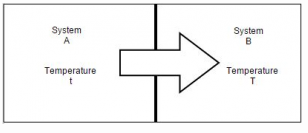# Test: Modes Of Heat Transfer

## 10 Questions MCQ Test Heat Transfer for Engg. | Test: Modes Of Heat Transfer

Description
This mock test of Test: Modes Of Heat Transfer for Chemical Engineering helps you for every Chemical Engineering entrance exam. This contains 10 Multiple Choice Questions for Chemical Engineering Test: Modes Of Heat Transfer (mcq) to study with solutions a complete question bank. The solved questions answers in this Test: Modes Of Heat Transfer quiz give you a good mix of easy questions and tough questions. Chemical Engineering students definitely take this Test: Modes Of Heat Transfer exercise for a better result in the exam. You can find other Test: Modes Of Heat Transfer extra questions, long questions & short questions for Chemical Engineering on EduRev as well by searching above.
QUESTION: 1

### The literature of heat transfer generally recognizes distinct modes of heat transfer. How many modes are there?

Solution:

There are three modes of heat transfer i.e. radiation, convection and conduction.

QUESTION: 2

### Consider system A at uniform temperature t and system B at another uniform temperature T (t > T). Let the two systems be brought into contact and be thermally insulated from their surroundings but not from each other. Energy will flow from system A to system B because ofSolution:

Greater the temperature imbalance the higher would be the rate of energy transfer.

QUESTION: 3

### An oil cooler in a high performance engine has an outside surface area 0.12 m2 and a surface temperature of 65 degree Celsius. At any intermediate time air moves over the surface of the cooler at a temperature of 30 degree Celsius and gives rise to a surface coefficient equal to 45.4 W/ m 2 K. Find out the heat transfer rate?

Solution:

: Q = (T2 – T1) A h = 0.12 (65-30) 45.4 = 190.68 W.

QUESTION: 4

Unit of rate of heat transfer is

Solution:

Unit of heat transfer is Joule but rate of heat transfer is joule per second i.e. watt.

QUESTION: 5

Convective heat transfer coefficient doesn’t depend on

Solution:

It is denoted by h and is dependent on space, time, geometry, orientation of solid surface.

QUESTION: 6

The rate equation used to describe the mechanism of convection is called Newton’s law of cooling. So rate of heat flow by convection doesn’t depend on

Solution:

It is directly proportional to all of above except time.

QUESTION: 7

How many types of convection process are there?

Solution:

Forced, natural and mixed convection.

QUESTION: 8

Thermal conductivity is maximum for which substance

Solution:

Thermal conductivity of diamond is 2300 W/m K.

QUESTION: 9

A radiator in a domestic heating system operates at a surface temperature of 60 degree Celsius. Calculate the heat flux at the surface of the radiator if it behaves as a black body

Solution:

As, q = Q/A

= 5.67 * 10-8 (273+60)4 = 697.2.

QUESTION: 10

Which of the following is an example of forced convection?

Solution:

In forced convection the flow of fluid is caused by a pump, fan or by atmospheric winds.